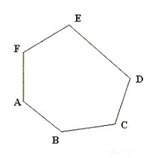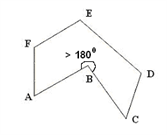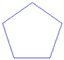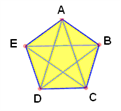# Polygons Introduction

As the CAT exam is one of the most competitive exams in the country, the MBA aspirants are required to be thoroughly prepared with all the important topics to ace the exam. So, here is a detailed explanation of one of the most crucial topics i.e. the Polygons.

## Polygons

A plane figure formed by three or more non-collinear points joined by line segments is called a polygon Polygons have special names, depending on the number of sides they have.

 Number of Sides Name of the Polygon 3 Triangle 4 Quadrilateral 5 Pentagon 6 Hexagon 7 Heptagon 8 Octagon 9 Nonagon 10 Decagon

## Types Of Polygons

1. Convex Polygon

A polygon in which none of the interior angles is more than 1800 is called a convex polygon2. Concave polygon

A polygon which has at least one interior angle > 1800 is called a concave polygon3. Regular polygon

A regular polygon is a polygon with equal sides and equal angles. It is a type of convex polygonOther definitions:

• 1) Perimeter➜ The perimeter of a figure is the sum of the lengths of all its sides
• 2) Area➜The region enclosed within a figure is called its area
• 3) Diagonal➜The segment joining any two non-consecutive vertices is called a diagonal.Here AD, BE, EC etc are all diagonals (Note:- Number of diagonals in a polygon can be found as ( nC2-n ) where n➜ number of sides in the polygon).

## Properties Of Polygons

• Sum of all interior angles of a regular polygon of side n is given by (2n-4)900. hence each angle of a regular polygon= ((2n-4) 900)/n
• Sum of an interior angle and adjacent exterior angle = 1800
• Sum of all exterior angles of a polygon= 3600

Hence, each exterior angle = 360/n (n➜ number of sides)

• Area of a regular polygon = ½ * (perimeter)*(perpendicular from center to any side)
• Number of diagonals in a polygon= nC2-n

If there are n sides in a polygon, there are “n” vertices as well.

To form a line we need 2 points which can be selected in nC2 ways

This includes all the diagonals as well as the sides of the polygon.

We thus need to remove the sides (n) Hence, the number of diagonals in a polygon= nC2-n

Examples:

1. A regular polygon with n sides has interior angles measuring 1740. what is the value of 180/n?

Solution:

Exterior angle= 180-174=60 . Here each exterior angle = 360/n. Hence, 180/n= 30

2. How many sides are there in a regular polygon which has the measure of one of its interior angles as 1770?

Solution:

Each Exterior angle = 180-177=30. Each exterior angle = 360/n= 3 => n=120.

The candidates are also suggested to learn all the important topics to properly prepare for the exam. Also, getting acquainted with the latest CAT 2017 notification is very crucial to strategize self-preparation.

To get more lessons on the important exam topics, visit Byju’s. Candidates can also find all the relevant information about the exam and the CAT registration process along with various CAT mocks and sample papers to help candidates ace the exam and get admissions in the top B-Schools of the nation.